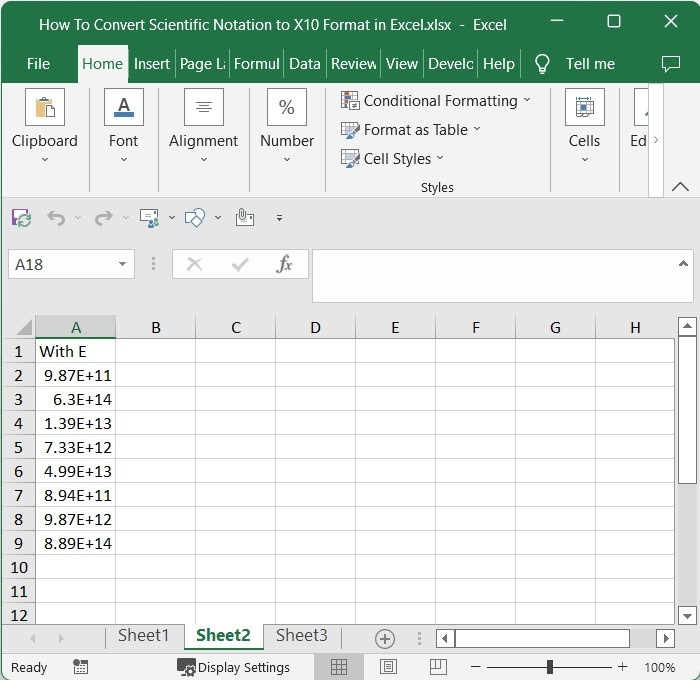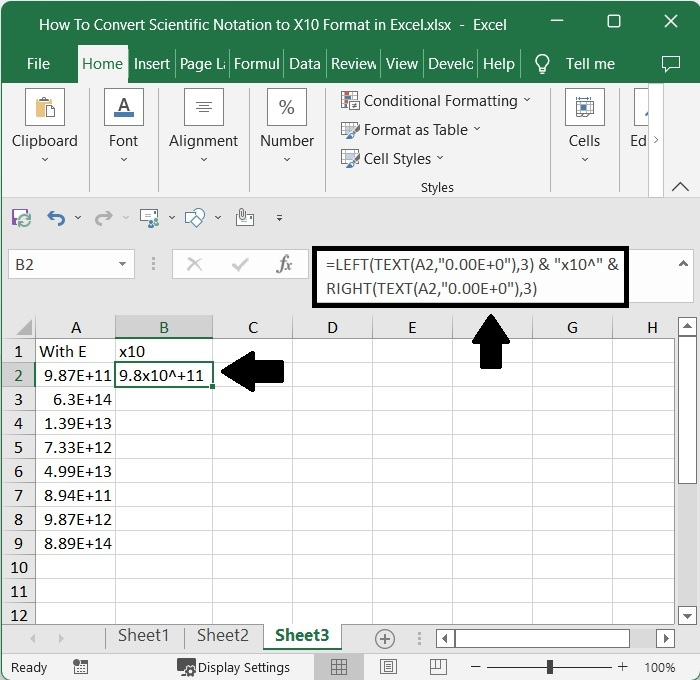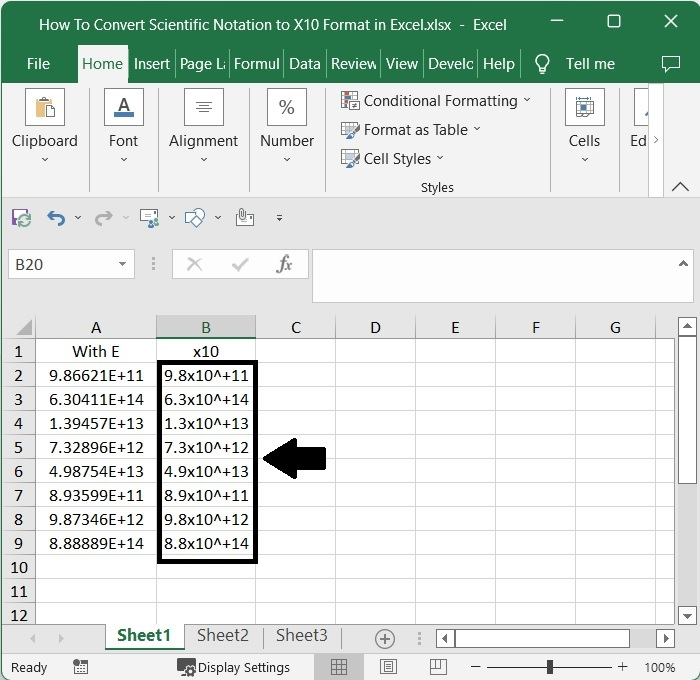# How to Convert Scientific Notation to X10 Format in Excel?

In Excel, most numbers with more than eleven digits are automatically converted to short form using the letter E, although scientific values may have more digits than eleven. Due to the fact that in mathematics, we always represent the short forms in multiples of 10, some people may find it difficult to understand what the letters E mean. Therefore, it is crucial for us to multiply the values with E by 10 in order to reach more customers. Manual conversion of those values can take a long time and result in values that are not accurate. The Excel formulas can be used to finish this task.

## Converting Scientific Notation to X10 Format in Excel

Here, we will first get any one of the results using the text formula and then get all the results using the Auto-fill handle. Let's take a look at a simple procedure for converting scientific notation to x10 format in Excel. We will be using the formula to complete our task.

### Step 1

Consider an Excel sheet with a list of scientific notations represented by the letter E, as shown in the image below.Now, in cell B2, click on an empty cell and enter the formula as follows −

=LEFT(TEXT(A2,"0.00E+0"),3) &"x10"&RIGHT(TEXT(A2,"0.00E+0"),3) and click enter to get our first value, similar to the below image. In the formula, A2 is the address of our value on the sheet.

Enter > Formula > Blank cell### Step 2

Then drag down from the first result using the auto-fill handle till all the results are filled, and our final result will be similar to the below image.## Conclusion

In this tutorial, we used a simple example to demonstrate how we can convert scientific notation to X10 format in Excel.

Updated on: 06-Mar-2023

1K+ Views